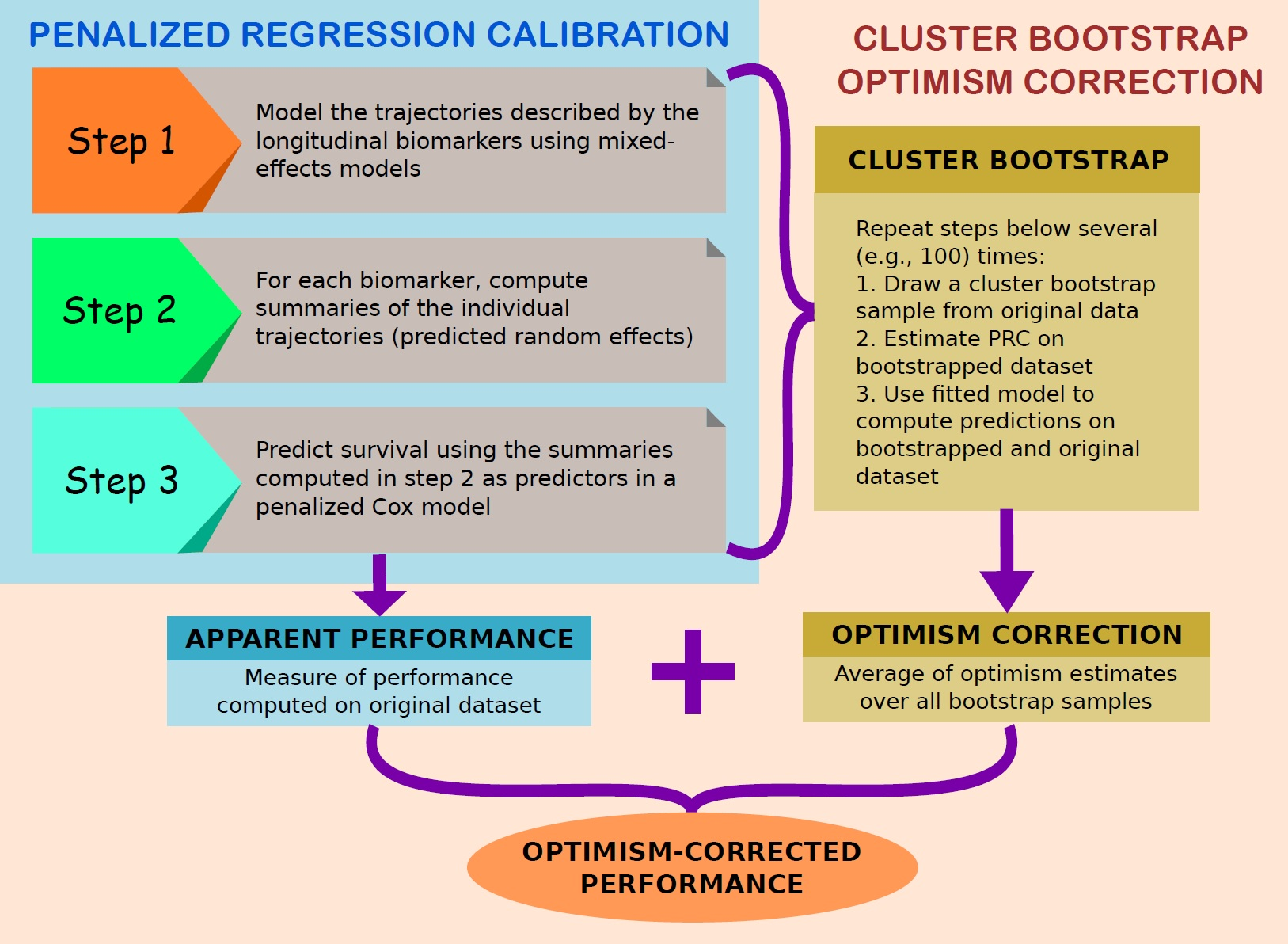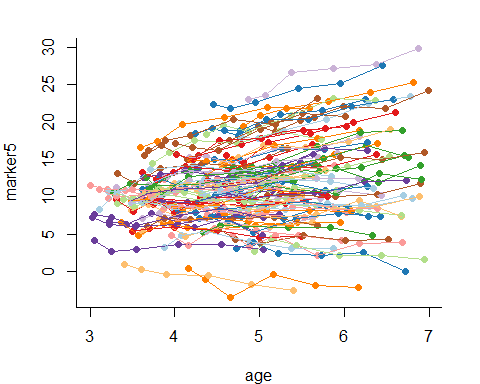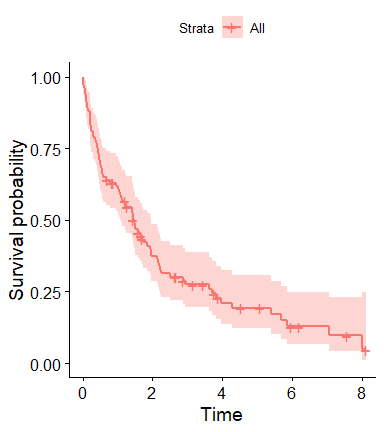# Introduction

## What is pencal?

pencal is an R package that has been created to make it easy and efficient to estimate and apply Penalized Regression Calibration (Signorelli et al., 2021).

## What is Penalized Regression Calibration?

Longitudinal and high-dimensional measurements have become increasingly common in biomedical research. However, methods to predict survival outcomes using covariates that are both longitudinal and high-dimensional are currently missing.

Penalized Regression Calibration (PRC) is a statistical method that has been developed to fill this methodological gap, making it possible to predict survival using as predictors a set of covariates that are at the same time longitudinal and high-dimensional.

PRC is described in detail in the following article:

Signorelli, M., Spitali, P., Al-Khalili Sgyziarto, C., The Mark-MD Consortium, Tsonaka, R. (2021). Penalized regression calibration: a method for the prediction of survival outcomes using complex longitudinal and high-dimensional data. arXiv preprint: arXiv:2101.04426.

## How does PRC work?

In short, PRC comprises three modelling steps:

1. in the first step, we model the trajectories described by the longitudinal biomarkers using mixed effects models;

2. in the second step, we compute subject-specific summaries of the longitudinal trajectories. In practice, these summaries are the predicted random effects from the mixed models estimated in step 1, and they allow us to summarize the way in which the biomarkers change over time across subjects;

3. in the third step, we estimate a penalized Cox model where the summaries computed in step 3 (alongside with other relevant covariates, such as baseline age or important confounders) are employed as predictors of the survival time.

By performing these 3 steps we can estimate PRC, obtaining a model that allows us to compute predicted survival probabilities.

Additionally, one may want to quantify the predictive performance of the fitted model. To achieve this aim, in pencal we have implemented a Cluster Bootstrap Optimism Correction Procedure (CBOCP) that can be used to obtain optimism-corrected estimates of the C index and time-dependent AUC associated to the fitted model. Depending on the dimensionality of your dataset, computing the CBOCP might be time consuming; for this reason, we offer the possibility to parallelize the CBOCP using multiple cores.

Below you can see a graphical representation of the steps involved in the estimation of PRC (see the elements in the lightblue box) and in the computation of the CBOCP (elements in the salmon box).## Get started

The R package pencal can be installed directly from CRAN using

install.packages('pencal')

After installing pencal, you can load the package with

library('pencal')

# Overview of the functionalities of pencal

Currently, pencal contains functions to estimate the PRC-LMM and PRC-MLPMM models proposed in Signorelli et al. (2021). An overview of the different functions is presented in the following subsections.

## Model estimation and prediction

Estimation of the PRC-LMM model can be performed by running sequentially the following three functions:

1. fit_lmms, which implements the first step of the estimation of the PRC-LMM;
2. summarize_lmms, which carries out the second step;
3. fit_prclmm, which performs the third step.

As already mentioned, these functions have to be run sequentially, with the output of fit_lmms used as input for summarize_lmms, and the output of summarize_lmms as input for fit_prclmm.

Similarly, estimation of the PRC-MLPMM model can be performed using:

1. fit_mlpmms, which implements the first step of the estimation of the PRC-MLPMM;
2. summarize_mlpmms, which carries out the second step;
3. fit_prcmlpmpm, which performs the third step.

## Predicted survival probabilities

Computation of the predicted survival probabilities from the PRC-LMM and PRC-MLPMM models can be done with the survpred_prc function.

## Predictive performance and cluster bootstrap optimism correction procedure (CBOCP)

The evaluation of the predicted performance of the estimated PRC-LMM and PRC-MLPMM can be done using the function performance_prc, which returns the naive and optimism-corrected estimates of the C index and of the time-dependent AUC. The optimism-corrected estimates are based on the CBOCP proposed in Signorelli et al. (2021).

A technical note on how the CBOCP is implemented in pencal: most of the computations required by the CBOCP are performed by fit_lmms, summarize_lmms and fit_prclmm for the PRC-LMM, and by fit_mlpmms, summarize_mlpmms and fit_prcmlpmm for the PRC-MLPMM. Such computations may be time-consuming, and for this reason these functions are designed to work with parallel computing (this can be easily done by setting the argument n.cores to a value $$> 1$$). The last step of the CBOCP is performed by the function performance_prc, which aggregates the outputs of the previous functions and computes the naive and optimism-corrected estimates of the C index and of the time-dependent AUC.

Important note: if you just want to estimate the PRC model, and you do not wish to compute the CBOCP, simply set n.boots = 0 as argument of fit_lmms. If, instead, you do want to compute the CBOCP, set n.boots equal to the desired number of bootstrap samples (e.g., 100).

## Further functions

In addition to the functions mentioned above, pencal comprises also 3 functions that can be used to simulate example datasets:

• simulate_t_weibull to simulate survival data from a Weibull model;
• simulate_prclmm_data to simulate an example dataset for PRC-LMM that is comprehensive of a number of longitudinal biomarkers, a survival outcome and a censoring indicator;
• simulate_prcmlpmm_data to simulate an example dataset for PRC-MLPMM.

# A step by step example

To illustrate how pencal works, let us simulate an example dataset from the PRC-LMM model. Hereafter we generate a dataset that comprises $$n = 100$$ subjects, $$p = 10$$ longitudinal biomarkers that are measured at $$t = 0, 0.2, 0.5, 1, 1.5, 2$$ years from baseline, and a survival outcome that is associated with 5 (p.relev) of the 10 biomarkers:

set.seed(1234)
p = 10
simdata = simulate_prclmm_data(n = 100, p = p, p.relev = 5,
lambda = 0.2, nu = 1.5,
seed = 1234, t.values = c(0, 0.2, 0.5, 1, 1.5, 2))
ls(simdata)
##  "censoring.prop" "long.data"      "surv.data"

Note that in this example we are setting $$n > p$$, but pencal can handle both low-dimensional ($$n > p$$) and high-dimensional ($$n \leq p$$) datasets.

## Input data

In order to estimate the PRC-LMM, you need to provide the following two inputs:

1. a dataset in long format, which should contain (at least) the following variables: a subject identifier that should be named id, the longitudinal biomarkers (here called marker1, …, marker10), and the relevant time variables (in this example we will use age as covariate in the LMMs estimated in step 1, and baseline.age as covariate in the penalized Cox model estimated in step 3):
# view the dataset in long format
head(simdata$long.data) ## id base.age t.from.base age marker1 marker2 marker3 marker4 ## 1 1 4.269437 0.0 4.269437 1.5417408 4.452282 15.13419 5.809207 ## 2 1 4.269437 0.2 4.469437 1.2346437 5.252873 15.98066 5.896591 ## 3 1 4.269437 0.5 4.769437 2.0773929 3.714174 18.44501 6.634897 ## 4 1 4.269437 1.0 5.269437 0.2137868 4.092887 20.23194 6.179779 ## 5 1 4.269437 1.5 5.769437 1.3354611 5.032044 18.99531 5.914160 ## 6 1 4.269437 2.0 6.269437 0.7811953 4.946483 22.19621 5.981212 ## marker5 marker6 marker7 marker8 marker9 marker10 ## 1 13.62807 -7.041495 15.19982 10.12011 2.7166023 15.16749 ## 2 15.03320 -5.763194 16.25356 10.20624 1.2764132 13.11855 ## 3 14.90197 -6.478355 17.40369 11.74692 1.9369628 13.91899 ## 4 16.48330 -8.994558 18.44549 11.91967 1.5949944 15.50285 ## 5 16.38560 -9.034169 19.61104 12.59247 1.3730259 15.86172 ## 6 17.23651 -10.220797 19.71013 12.79891 -0.2164965 15.89041 # visualize the trajectories for a randomly picked biomarker library(ptmixed) ptmixed::make.spaghetti(x = age, y = marker5, id = id, group = id, data = simdata$long.data,
margins = c(4, 4, 2, 2),
legend.inset = - 1)1. a dataset with information on the survival outcome, which should contain (at least) the following variables: a subject identifier that should be named id, the time to event outcome called time, and the binary event indicator called event (NB: make sure that the variable names associated to these three variables are indeed id, time and event!)
# view the dataset with the survival data
head(simdata$surv.data) ## id baseline.age time event ## 1 1 4.269437 0.8368389 0 ## 2 2 4.705434 1.4288656 1 ## 3 3 3.220979 1.6382975 1 ## 4 4 4.379400 0.5809532 1 ## 5 5 4.800329 0.2441706 1 ## 6 6 3.394879 0.4901404 1 # what is the proportion of censoring in this dataset? simdata$censoring.prop
##  0.22
# visualize an estimate of the survival function
library(survival)
library(survminer)
## Loading required package: ggplot2
## Loading required package: ggpubr
surv.obj = survival::Surv(time = simdata$surv.data$time,
event = simdata$surv.data$event)
kaplan = survival::survfit(surv.obj ~ 1,
type="kaplan-meier")
survminer::ggsurvplot(kaplan, data = simdata$surv.data)## Some remarks on parallel computing with pencal Before we begin to estimate the PRC-LMM, let’s determine the number of cores that will be used for the computation of the CBOCP. In general you can use as many cores as available to you; to do this, you can set n.cores = parallel::detectCores() Since the CRAN Repository Policy allow us to use at most 2 cores when building the vignettes, in this example we will limit the number of cores used to 2: n.cores = 2 Be aware, however, that using more than 2 cores will speed computations up, and it is thus recommended. Several functions in pencal will actually return a warning when you perform computations using less cores than available: the goal of such warnings is to remind you that you could use more cores to speed computations up; however, if you are purposedly using a smaller number of cores you can ignore the warning. ## Estimation of the PRC-LMM Hereafter we show how to implement the three steps involved in the estimation of the PRC-LMM, alongside with the computation of the CBOCP. ### Step 1 In the first step, for each biomarker we estimate a linear mixed model (LMM) where the longitudinal biomarker levels $$y_{ij}$$ depend on two fixed effects (one intercept, $$\beta_0$$ and one slope for age, $$\beta_1$$), on a subject-specific random intercept $$u_{0i}$$ and on a random slope for age $$u_{1i}$$: $y_{ij} = \beta_0 + u_{0i} + \beta_1 a_{ij} + u_{1i} a_{ij} + \varepsilon_{ij}.$ To do this in R we use the fit_lmms function: y.names = paste('marker', 1:p, sep = '') step1 = fit_lmms(y.names = y.names, fixefs = ~ age, ranefs = ~ age | id, long.data = simdata$long.data,
surv.data = simdata$surv.data, t.from.base = t.from.base, n.boots = 10, n.cores = n.cores) ## Sorting long.data by subject id ## Sorting surv.data by subject id ## Preliminary step: remove measurements taken after event / censoring. ## Removed: 209 measurements. Retained: 391 measurements. ## Estimating the LMMs on the original dataset... ## ...done ## Bootstrap procedure started ## This computation will be run in parallel, using 2 cores ## Bootstrap procedure finished ## Computation of step 1: finished :) The LMM fitted here is just an example of how to model longitudinal biomarker trajectories: depending on the data you are dealing with, you may choose to specify different fixed and random effects formulas, or even to consider the MLPMM instead of the LMM. Note that here I have set n.boots = 10 to reduce computing time for the CBOCP, given that CRAN only allows me to use two cores when compiling the vignette. In general, it is recommended to set n.boots = 0 if you do not wish to compute the CBOCP, or to set n.boots equal to a larger number (e.g., 50, 100 or 200) if you want to accurately compute the CBOCP. In the latter case, consider using as many cores as available to you to speed computations up. fit_lmms returns as output a list with several elements; among them is lmm.fits.orig, which contains the LMMs fitted to each biomarker: ls(step1) ##  "boot.ids" "call.info" "df.sanitized" "lmm.fits.boot" ##  "lmm.fits.orig" "n.boots" # estimated LMM for marker1: step1$lmm.fits.orig
## $marker1 ## Linear mixed-effects model fit by REML ## Data: df.sub ## Log-restricted-likelihood: -678.8922 ## Fixed: fixef.formula ## (Intercept) age ## 3.491217 -1.025154 ## ## Random effects: ## Formula: ~age | id ## Structure: General positive-definite, Log-Cholesky parametrization ## StdDev Corr ## (Intercept) 1.4809966 (Intr) ## age 0.8241438 0.391 ## Residual 0.7262114 ## ## Number of Observations: 391 ## Number of Groups: 100 For more details about the arguments of fit_lmms and its outputs, see the help page: ?fit_lmms. ### Step 2 In the second step we compute the predicted random intercepts and random slopes for the LMMs fitted in step 1: step2 = summarize_lmms(object = step1, n.cores = n.cores) ## Computing the predicted random effects on the original dataset... ## ...done ## Bootstrap procedure started ## This computation will be run in parallel, using 2 cores ## Bootstrap procedure finished ## Computation of step 2: finished :) summarize_lmms returns as output a list that contains, among other elements, a matrix ranef.orig with the predicted random effects for the LMMs fitted in step 1: ls(step2) ##  "boot.ids" "call" "n.boots" "ranef.boot.train" ##  "ranef.boot.valid" "ranef.orig" # view predicted random effects for the first two markers step2$ranef.orig[1:5, 1:4]
##   marker1_b_int marker1_b_age marker2_b_int marker2_b_age
## 1   0.468791151    0.50257974     0.4806176     0.9897538
## 2  -0.651187418   -1.18949489     0.3094290    -1.2798246
## 3   0.541710892    1.35639622    -1.1855801    -0.4835886
## 4   1.065842498    0.82801424    -0.4332657     0.1949000
## 5   0.002714736   -0.09192979    -0.4224219    -1.4788431

For more details about the arguments of summarize_lmms and its outputs, see the help page: ?summarize_lmms.

### Step 3

Lastly, in the third step of PRC-LMM we estimate a penalized Cox model where we employ as predictors baseline age and all the summaries (predicted random effects) computed in step 2:

step3 = fit_prclmm(object = step2, surv.data = simdata$surv.data, baseline.covs = ~ baseline.age, penalty = 'ridge', n.cores = n.cores) ## Estimated penalized Cox model on the original dataset... ## ...done ## Bootstrap procedure started ## This computation will be run in parallel, using 2 cores ## Bootstrap procedure finished ## Computation of step 3: finished :) In this example I have set penalty = 'ridge', but you may also use elasticnet or lasso as alternatives. Moreover, by default the predicted random effects are standardized when included in the penalized Cox model (if you don’t want to perform such standardization, set standardize = F). fit_prclmm returns as output a list that contains, among other elements, the fitted penalized Cox model pcox.orig, which is a glmnet object: ls(step3) ##  "boot.ids" "call" "n.boots" "pcox.boot" "pcox.orig" "surv.data" class(step3$pcox.orig)
##  "cv.glmnet"
library(glmnet)
## Loading required package: Matrix
## Loaded glmnet 4.0-2
t(as.matrix(coef(step3$pcox.orig))) ## baseline.age marker1_b_int marker1_b_age marker2_b_int marker2_b_age ## 1 0.001253804 -0.02091294 -0.005629194 0.05632732 0.1530983 ## marker3_b_int marker3_b_age marker4_b_int marker4_b_age marker5_b_int ## 1 0.103437 0.06736506 -0.0103572 -0.0005451742 -0.3558578 ## marker5_b_age marker6_b_int marker6_b_age marker7_b_int marker7_b_age ## 1 -0.074645 2.170329 0.02189938 0.03473504 0.01001565 ## marker8_b_int marker8_b_age marker9_b_int marker9_b_age marker10_b_int ## 1 -0.3016531 0.02321143 -0.1515156 -0.03053159 0.02916538 ## marker10_b_age ## 1 0.001877107 For more details about the arguments of fit_prclmm and its outputs, see the help page: ?fit_prclmm. ## Predicted survival probabilities After fitting the model, you will probably want to obtain predicted survival probabilities for each individual at several time points. This can be done through the function survpred_prc, which takes as inputs the outputs of step 2 and step 3, alongside with the time points at which to compute the survival probabilities: preds = survpred_prc(step2, step3, times = c(1, 2, 3)) head(preds) ## id S(1) S(2) S(3) ## 1 1 0.4685893 0.1666528 0.08151238 ## 2 2 0.7506514 0.5076432 0.38729468 ## 3 3 0.7088022 0.4432699 0.32036642 ## 4 4 0.5153831 0.2087025 0.11167056 ## 5 5 0.6207733 0.3239896 0.20662124 ## 6 6 0.5630938 0.2572870 0.14965965 ## Cluster bootstrap optimism correction and performance measures To accurately quantify the predicted performance of the fitted PRC model, we need to recur to some form of internal validation strategy (e.g., bootstrap, cross-validation, etc…). In pencal the internal validation is performed through a Cluster Bootstrap Optimism Correction Procedure (CBOCP) that allows to compute optimism-corrected estimates of the concordance (C) index and of the time-dependent AUC. Most of the steps that the CBOCP requires are directly computed by the functions fit_lmms, summarize_lmms and fit_prclmm whenever the argument n.boots of fit_lmms is set equal to an integer > 0 (in other words: most of the computations needed for the CBOCP have already been performed in the code chunks executed above, so we are almost done!). To gather the results of the CBOCP we can use the function performance_prc: cbocp = performance_prc(step2, step3, times = c(1, 2, 3), n.cores = n.cores) ## Computation of optimism correction started ## This computation will be run in parallel, using 2 cores ## Computation of the optimism correction: finished :) # C index estimates: cbocp$concordance
##   n.boots C.naive cb.opt.corr C.adjusted
## 1      10  0.7855     -0.0397     0.7458
# time-dependent AUC estimates:
cbocp\$tdAUC
##   pred.time tdAUC.naive cb.opt.corr tdAUC.adjusted
## 1         1      0.8874     -0.0386         0.8488
## 2         2      0.8337     -0.0442         0.7895
## 3         3      0.8303     -0.0674         0.7629

From the results above we can see that:

• the naive C index is estimated = 0.786, and the optimism-corrected C index is estimated = 0.747;
• the naive tdAUC for predictions at 1 year from baseline is estimated = 0.887, and the optimism-corrected estimate is = 0.850;
• the naive tdAUC for predictions at 2 years from baseline is estimated = 0.834, and the optimism-corrected estimate is = 0.796;
• the naive tdAUC for predictions at 3 years from baseline is estimated = 0.830, and the optimism-corrected estimate is = 0.770.

# Further details and material

The aim of this vignette is to provide a quick-start introduction to the R package pencal. Here I have focused my attention on the fundamental aspects that one needs to use the package.

Further details, functions and examples can be found in the manual of the package.

The description of the method is available in an article that you can read here.